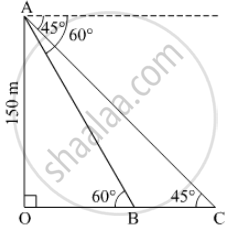# A Moving Boat is Observed from the Top of a 150 M High Cliff Moving Away from the Cliff. the Angle of Depression of the Boat Changes from 60° to 45° in 2 Minutes. Find the Speed of the Boat in M/Min. - Mathematics

Sum

A moving boat is observed from the top of a 150 m high cliff moving away from the cliff. The angle of depression of the boat changes from 60° to 45° in 2 minutes. Find the speed of the boat in m/min.

#### SolutionLet AO be the cliff of height 150 m.
Let the speed of the boat be x meters per minute.
And BC is the distance which man travelled.

So, BC = 2x         ....[ ∵Distance = Speed x Time ]

tan(60°) = "AO"/"OB"

sqrt3 = 150/"OB"

⇒ OB = (150sqrt3)/3 = 50sqrt3

tan(45°) = "AO"/"OC"

⇒1 = 150/"OC"

⇒ OC = 150
Now OC = OB + BC

⇒ 150 = 50sqrt3 + 2x

⇒ x = (150 − 50sqrt3)/2

⇒ x = 75 − 25sqrt3

Using sqrt3 = 1.73
x = 75 − 25 x 1.732 ≈ 32 m/min
Hence, the speed of the boat is 32 metres per minute.

Is there an error in this question or solution?